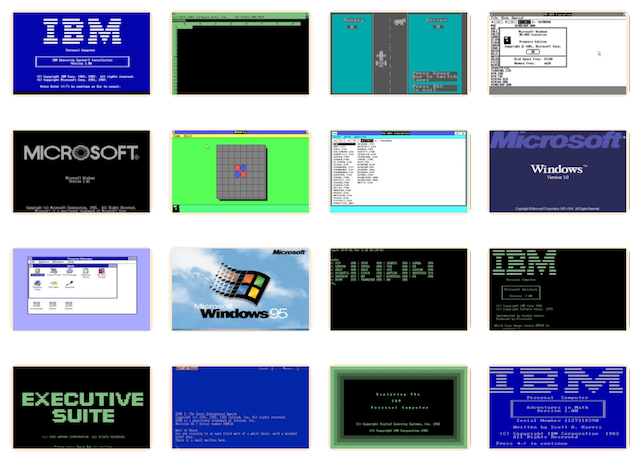# PCjs Machines

Home of the original IBM PC emulator for browsers.## PC-SIG Diskette Library (Disk #394)

[PCjs Machine "ibm5170"]

Waiting for machine "ibm5170" to load....

## Information about “MATH PAK III”

``````MATH PAK III is a set of tutorials to help improve your problem-solving
skills plus mathematical conversion routines to speed up some math
operations.

It contains a range of tutorials -- from the very basic arithmetic
functions of addition, subtraction, multiplication and division to
solving quadratic equations, computing geometries and understanding trig
functions.  MATH PAK III will also read your own data files to solve
quadratic equations, derivatives, linear equations, DEC-BIN-HEX-OCT
conversions, trig functions, determinants and more.

It offers 80x87 math coprocessor support, context-sensitive help
screens, fast execution and printing of trig tables and sample problems
to solve.  Registration includes a 50 page manual with examples and
solved problems.

This set of programs can be used as an educational program to help
expand your knowledge and appreciation of mathematics.
``````

## FILE0394.TXT

``````Disk No:  394
Disk Title: Math Pak III
PC-SIG Version: S4.1

Program Title: MATH PAK III
Author Version: 1.5A
Author Registration: \$9.95
Special Requirements: None.

MATH PAK II is a set of tutorials to help improve your problem-solving
skills and mathematical conversion routines to speed up some math
operations.

A faculty of tutorials -- from the very basic arithmetic functions of
equations, computing geometries and understanding trig functions.
Included are conversion tables on ASCII-Decimal-Hex and Metric-English
translations.

This set of programs can be used as an educational program to help
expand your knowledge and appreciation of mathematics.

PC-SIG
1030D East Duane Avenue
Sunnyvale  Ca. 94086
(408) 730-9291
``````

## GO.TXT

``````╔═════════════════════════════════════════════════════════════════════════╗
║                  <<<<  Disk #394 MATH PAK III  >>>>                     ║
╠═════════════════════════════════════════════════════════════════════════╣
║ To view the documentation type: README  (press enter)                   ║
║                                                                         ║
║ To Start the program type: MPK3  (press enter)                          ║
╚═════════════════════════════════════════════════════════════════════════╝
``````

## MPK3.DOC

``````
MATH PAK III v1.0 (C) 1990 Dalal Publishing Co.

MATH PAK III v1.0 offers the following options to the PC user:

-automatic 80x87 support
-clear, consise help screens, with context sensitive help using the F1 key
-standalone program, in .EXE form. Needs no other software or compilers to run
-decimal-hexidecimal-binary-octal base conversions
-chain rule solving for derivatives
-first and second derivative solving for polynomials
-word problems
-linear equation solving
-statistical functions, including mean, standard deviation,median
-polynomial intersections solutions
-two and three variable equation solving, using Cramer's rule
-solutions to a line, given two points on the line
-trig functions
-much more !

Also included with the MATH PAK III main program (MPK3.EXE), are these three
programs/utilities:

MATH PAK III Data File Utility - This program allows the user to use his own
ASCII data files to solve for quadratic equations, linear equations and first
and second derivative solutions.

MATH PAK III Problem Solving Utility - This program allows the user to print
out to an on-line printer, selected problems to work out. These include problems
in base conversions, additions, subtraction, multiplication, fractions, word
problems, a trig table and much more. NEEDS 80x87 chip to work.

MATH PAK III Test File Utility - This program will test your ASCII data files
for proper syntax, in terms of alpha characters, etc. and tell you if the
file will be compatable with the MATH PAK III Data File Utility.

Registration: US \$9.95 plus US \$ 3.00 for shipping and handling. You will
receive a 50+ page manual, showing examples, solved problems and how to use
the programs to their full potential.

Contact Dalal Publishing Co., at (408) 736 - 4744(9:00am to 5:00pm, PST)

Or write :

Dalal Publishing Co.
180 Elm Ct. # 1503
Sunnyvale, CA 94086


``````

### Directory of PC-SIG Library Disk #0394

`````` Volume in drive A has no label
Directory of A:\

MPK3DATA EXE     42288   4-12-90  12:01a
MPK3PROB COM     25344   4-12-90  12:01a
MPK3TF   EXE      7744   4-12-90  12:01a